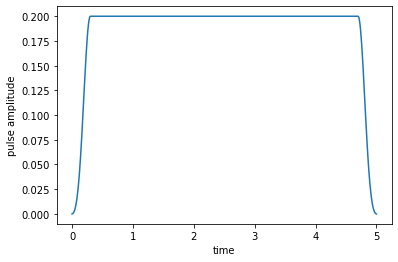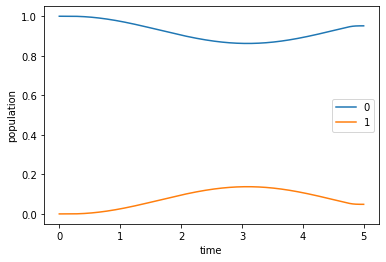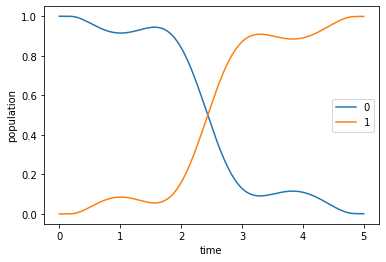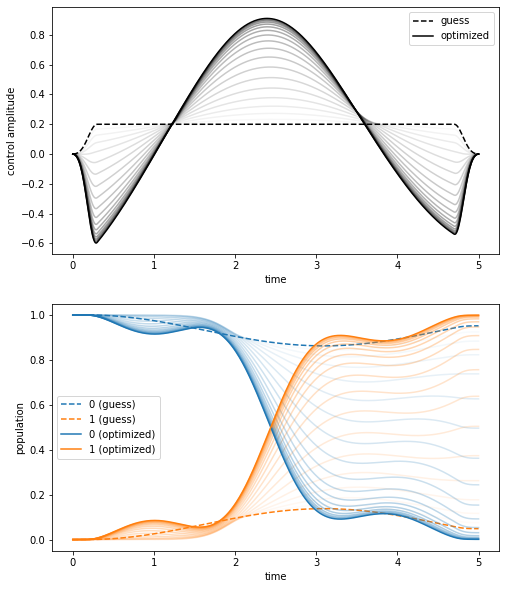# Optimization of a State-to-State Transfer in a Two-Level-System¶

:

# NBVAL_IGNORE_OUTPUT
import qutip
import numpy as np
import scipy
import matplotlib
import matplotlib.pylab as plt
import krotov
%watermark -v --iversions

matplotlib       3.1.2
matplotlib.pylab 1.17.2
scipy            1.3.1
numpy            1.17.2
qutip            4.4.1
krotov           1.0.0
CPython 3.7.3
IPython 7.10.2


$$\newcommand{tr}{\operatorname{tr}} \newcommand{diag}{\operatorname{diag}} \newcommand{abs}{\operatorname{abs}} \newcommand{pop}{\operatorname{pop}} \newcommand{aux}{\text{aux}} \newcommand{opt}{\text{opt}} \newcommand{tgt}{\text{tgt}} \newcommand{init}{\text{init}} \newcommand{lab}{\text{lab}} \newcommand{rwa}{\text{rwa}} \newcommand{bra}{\langle#1\vert} \newcommand{ket}{\vert#1\rangle} \newcommand{Bra}{\left\langle#1\right\vert} \newcommand{Ket}{\left\vert#1\right\rangle} \newcommand{Braket}{\left\langle #1\vphantom{#2}\mid{#2}\vphantom{#1}\right\rangle} \newcommand{op}{\hat{#1}} \newcommand{Op}{\hat{#1}} \newcommand{dd}{\,\text{d}} \newcommand{Liouville}{\mathcal{L}} \newcommand{DynMap}{\mathcal{E}} \newcommand{identity}{\mathbf{1}} \newcommand{Norm}{\lVert#1\rVert} \newcommand{Abs}{\left\vert#1\right\vert} \newcommand{avg}{\langle#1\rangle} \newcommand{Avg}{\left\langle#1\right\rangle} \newcommand{AbsSq}{\left\vert#1\right\vert^2} \newcommand{Re}{\operatorname{Re}} \newcommand{Im}{\operatorname{Im}}$$

This first example illustrates the basic use of the krotov package by solving a simple canonical optimization problem: the transfer of population in a two level system.

## Two-level-Hamiltonian¶

We consider the Hamiltonian $$\op{H}_{0} = - \frac{\omega}{2} \op{\sigma}_{z}$$, representing a simple qubit with energy level splitting $$\omega$$ in the basis $$\{\ket{0},\ket{1}\}$$. The control field $$\epsilon(t)$$ is assumed to couple via the Hamiltonian $$\op{H}_{1}(t) = \epsilon(t) \op{\sigma}_{x}$$ to the qubit, i.e., the control field effectively drives transitions between both qubit states.

:

def hamiltonian(omega=1.0, ampl0=0.2):
"""Two-level-system Hamiltonian

Args:
omega (float): energy separation of the qubit levels
ampl0 (float): constant amplitude of the driving field
"""
H0 = -0.5 * omega * qutip.operators.sigmaz()
H1 = qutip.operators.sigmax()

def guess_control(t, args):
return ampl0 * krotov.shapes.flattop(
t, t_start=0, t_stop=5, t_rise=0.3, func="blackman"
)

return [H0, [H1, guess_control]]


:

H = hamiltonian()


The control field here switches on from zero at $$t=0$$ to it’s maximum amplitude 0.2 within the time period 0.3 (the switch-on shape is half a Blackman pulse). It switches off again in the time period 0.3 before the final time $$T=5$$). We use a time grid with 500 time steps between 0 and $$T$$:

:

tlist = np.linspace(0, 5, 500)

:

def plot_pulse(pulse, tlist):
fig, ax = plt.subplots()
if callable(pulse):
pulse = np.array([pulse(t, args=None) for t in tlist])
ax.plot(tlist, pulse)
ax.set_xlabel('time')
ax.set_ylabel('pulse amplitude')
plt.show(fig)

:

plot_pulse(H, tlist)## Optimization target¶

The krotov package requires the goal of the optimization to be described by a list of Objective instances. In this example, there is only a single objective: the state-to-state transfer from initial state $$\ket{\Psi_{\init}} = \ket{0}$$ to the target state $$\ket{\Psi_{\tgt}} = \ket{1}$$, under the dynamics of the Hamiltonian $$\op{H}(t)$$:

:

objectives = [
krotov.Objective(
initial_state=qutip.ket("0"), target=qutip.ket("1"), H=H
)
]

objectives

:

[Objective[|Ψ₀(2)⟩ to |Ψ₁(2)⟩ via [H₀[2,2], [H₁[2,2], u₁(t)]]]]


In addition, we would like to maintain the property of the control field to be zero at $$t=0$$ and $$t=T$$, with a smooth switch-on and switch-off. We can define an “update shape” $$S(t) \in [0, 1]$$ for this purpose: Krotov’s method will update the field at each point in time proportionally to $$S(t)$$; wherever $$S(t)$$ is zero, the optimization will not change the value of the control from the original guess.

:

def S(t):
"""Shape function for the field update"""
return krotov.shapes.flattop(
t, t_start=0, t_stop=5, t_rise=0.3, t_fall=0.3, func='blackman'
)


Beyond the shape, Krotov’s method uses a parameter $$\lambda_a$$ for each control field that determines the overall magnitude of the respective field in each iteration (the smaller $$\lambda_a$$, the larger the update; specifically, the update is proportional to $$\frac{S(t)}{\lambda_a}$$). Both the update-shape $$S(t)$$ and the $$\lambda_a$$ parameter must be passed to the optimization routine as “pulse options”:

:

pulse_options = {
H: dict(lambda_a=5, update_shape=S)
}


## Simulate dynamics under the guess field¶

Before running the optimization procedure, we first simulate the dynamics under the guess field $$\epsilon_{0}(t)$$. The following solves equation of motion for the defined objective, which contains the initial state $$\ket{\Psi_{\init}}$$ and the Hamiltonian $$\op{H}(t)$$ defining its evolution. This delegates to QuTiP’s usual mesolve function.

We use the projectors $$\op{P}_0 = \ket{0}\bra{0}$$ and $$\op{P}_1 = \ket{1}\bra{1}$$ for calculating the population:

:

proj0 = qutip.ket2dm(qutip.ket("0"))
proj1 = qutip.ket2dm(qutip.ket("1"))

:

guess_dynamics = objectives.mesolve(tlist, e_ops=[proj0, proj1])


The plot of the population dynamics shows that the guess field does not transfer the initial state $$\ket{\Psi_{\init}} = \ket{0}$$ to the desired target state $$\ket{\Psi_{\tgt}} = \ket{1}$$ (so the optimization will have something to do).

:

def plot_population(result):
fig, ax = plt.subplots()
ax.plot(result.times, result.expect, label='0')
ax.plot(result.times, result.expect, label='1')
ax.legend()
ax.set_xlabel('time')
ax.set_ylabel('population')
plt.show(fig)

:

plot_population(guess_dynamics)## Optimize¶

In the following we optimize the guess field $$\epsilon_{0}(t)$$ such that the intended state-to-state transfer $$\ket{\Psi_{\init}} \rightarrow \ket{\Psi_{\tgt}}$$ is solved, via the krotov package’s central optimize_pulses routine. It requires, besides the previously defined objectives, information about the optimization functional $$J_T$$ (implicitly, via chi_constructor, which calculates the states $$\ket{\chi} = \frac{J_T}{\bra{\Psi}}$$).

Here, we choose $$J_T = J_{T,\text{ss}} = 1 - F_{\text{ss}}$$ with $$F_{\text{ss}} = \Abs{\Braket{\Psi_{\tgt}}{\Psi(T)}}^2$$, with $$\ket{\Psi(T)}$$ the forward propagated state of $$\ket{\Psi_{\init}}$$. Even though $$J_T$$ is not explicitly required for the optimization, it is nonetheless useful to be able to calculate and print it as a way to provide some feedback about the optimization progress. Here, we pass as an info_hook the function krotov.info_hooks.print_table, using krotov.functionals.J_T_ss (which implements the above functional; the krotov library contains implementations of all the “standard” functionals used in quantum control). This info_hook prints a tabular overview after each iteration, containing the value of $$J_T$$, the magnitude of the integrated pulse update, and information on how much $$J_T$$ (and the full Krotov functional $$J$$) changes between iterations. It also stores the value of $$J_T$$ internally in the Result.info_vals attribute.

The value of $$J_T$$ can also be used to check the convergence. In this example, we limit the number of total iterations to 10, but more generally, we could use the check_convergence parameter to stop the optimization when $$J_T$$ falls below some threshold. Here, we only pass a function that checks that the value of $$J_T$$ is monotonically decreasing. The krotov.convergence.check_monotonic_error relies on krotov.info_hooks.print_table internally having stored the value of $$J_T$$ to the Result.info_vals in each iteration.

:

opt_result = krotov.optimize_pulses(
objectives,
pulse_options=pulse_options,
tlist=tlist,
propagator=krotov.propagators.expm,
chi_constructor=krotov.functionals.chis_ss,
info_hook=krotov.info_hooks.print_table(J_T=krotov.functionals.J_T_ss),
check_convergence=krotov.convergence.Or(
krotov.convergence.value_below('1e-3', name='J_T'),
krotov.convergence.check_monotonic_error,
),
store_all_pulses=True,
)

iter.      J_T    ∫gₐ(t)dt          J       ΔJ_T         ΔJ  secs
0     9.51e-01    0.00e+00   9.51e-01        n/a        n/a     1
1     9.24e-01    2.32e-03   9.27e-01  -2.70e-02  -2.47e-02     2
2     8.83e-01    3.53e-03   8.87e-01  -4.11e-02  -3.75e-02     2
3     8.23e-01    5.22e-03   8.28e-01  -6.06e-02  -5.54e-02     2
4     7.38e-01    7.39e-03   7.45e-01  -8.52e-02  -7.78e-02     2
5     6.26e-01    9.75e-03   6.36e-01  -1.11e-01  -1.01e-01     2
6     4.96e-01    1.16e-02   5.07e-01  -1.31e-01  -1.19e-01     2
7     3.62e-01    1.21e-02   3.74e-01  -1.34e-01  -1.22e-01     2
8     2.44e-01    1.09e-02   2.55e-01  -1.18e-01  -1.07e-01     2
9     1.53e-01    8.43e-03   1.62e-01  -9.03e-02  -8.19e-02     2
10    9.20e-02    5.80e-03   9.78e-02  -6.14e-02  -5.56e-02     2
11    5.35e-02    3.66e-03   5.72e-02  -3.85e-02  -3.48e-02     2
12    3.06e-02    2.19e-03   3.28e-02  -2.29e-02  -2.07e-02     2
13    1.73e-02    1.27e-03   1.86e-02  -1.33e-02  -1.20e-02     2
14    9.79e-03    7.24e-04   1.05e-02  -7.55e-03  -6.82e-03     2
15    5.52e-03    4.10e-04   5.93e-03  -4.27e-03  -3.86e-03     2
16    3.11e-03    2.31e-04   3.35e-03  -2.41e-03  -2.18e-03     2
17    1.76e-03    1.30e-04   1.89e-03  -1.36e-03  -1.23e-03     1
18    9.92e-04    7.36e-05   1.07e-03  -7.65e-04  -6.91e-04     1

:

opt_result

:

Krotov Optimization Result
--------------------------
- Started at 2019-12-15 22:36:37
- Number of objectives: 1
- Number of iterations: 18
- Reason for termination: Reached convergence: J_T < 1e-3
- Ended at 2019-12-15 22:37:16 (0:00:39)


## Simulate the dynamics under the optimized field¶

Having obtained the optimized control field, we can simulate the dynamics to verify that the optimized field indeed drives the initial state $$\ket{\Psi_{\init}} = \ket{0}$$ to the desired target state $$\ket{\Psi_{\tgt}} = \ket{1}$$.

:

opt_dynamics = opt_result.optimized_objectives.mesolve(
tlist, e_ops=[proj0, proj1])

:

plot_population(opt_dynamics)To gain some intuition on how the controls and the dynamics change throughout the optimization procedure, we can generate a plot of the control fields and the dynamics after each iteration of the optimization algorithm. This is possible because we set store_all_pulses=True in the call to optimize_pulses, which allows to recover the optimized controls from each iteration from Result.all_pulses. The flag is not set to True by default, as for long-running optimizations with thousands or tens of thousands iterations, the storage of all control fields may require significant memory.

:

def plot_iterations(opt_result):
"""Plot the control fields in population dynamics over all iterations.

This depends on store_all_pulses=True in the call to
optimize_pulses.
"""
fig, [ax_ctr, ax_dyn] = plt.subplots(nrows=2, figsize=(8, 10))
n_iters = len(opt_result.iters)
for (iteration, pulses) in zip(opt_result.iters, opt_result.all_pulses):
controls = [
krotov.conversions.pulse_onto_tlist(pulse)
for pulse in pulses
]
objectives = opt_result.objectives_with_controls(controls)
dynamics = objectives.mesolve(
opt_result.tlist, e_ops=[proj0, proj1]
)
if iteration == 0:
ls = '--'  # dashed
alpha = 1  # full opacity
ctr_label = 'guess'
pop_labels = ['0 (guess)', '1 (guess)']
elif iteration == opt_result.iters[-1]:
ls = '-'  # solid
alpha = 1  # full opacity
ctr_label = 'optimized'
pop_labels = ['0 (optimized)', '1 (optimized)']
else:
ls = '-'  # solid
alpha = 0.5 * float(iteration) / float(n_iters)  # max 50%
ctr_label = None
pop_labels = [None, None]
ax_ctr.plot(
dynamics.times,
controls,
label=ctr_label,
color='black',
ls=ls,
alpha=alpha,
)
ax_dyn.plot(
dynamics.times,
dynamics.expect,
label=pop_labels,
color='#1f77b4',  # default blue
ls=ls,
alpha=alpha,
)
ax_dyn.plot(
dynamics.times,
dynamics.expect,
label=pop_labels,
color='#ff7f0e',  # default orange
ls=ls,
alpha=alpha,
)
ax_dyn.legend()
ax_dyn.set_xlabel('time')
ax_dyn.set_ylabel('population')
ax_ctr.legend()
ax_ctr.set_xlabel('time')
ax_ctr.set_ylabel('control amplitude')
plt.show(fig)

:

plot_iterations(opt_result)The initial guess (dashed) and final optimized (solid) control amplitude and resulting dynamics are shown with full opacity, whereas the curves corresponding intermediate iterations are shown with decreasing transparency.